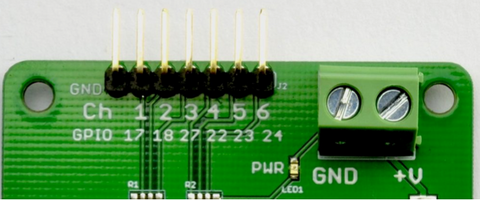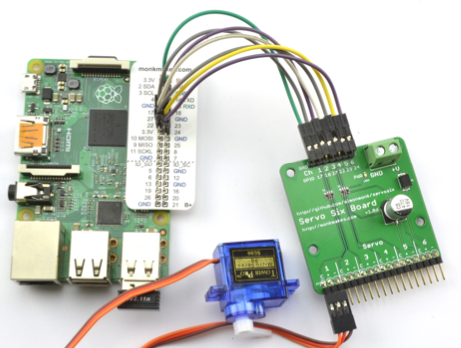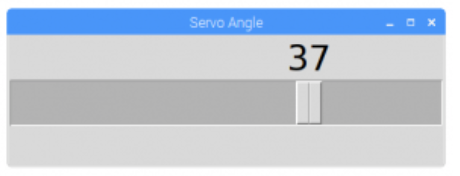Monk Makes

# ServoSix board

M059

\$6.95

20 items left

The Servo Six board simplifies the process of connecting up to six servo motors to a Raspberry Pi or Arduino.

The Servo Six has the following features:

• Screw terminals for servo power supply
• Reverse-polarity protection for the servo power supply
• 470μF 16V capacitor for servo supply
• 470Ω current limiting resistors for servo control lines (to protect GPIO pins)
• Power indicator LED

Connecting a Servo Six board to a Raspberry Pi

To connect a ServoSix board to a Raspberry Pi you need a female to female jumper wire to connect ground (GND) on the Raspberry Pi to GND on the ServoSix board. For each servo (up to six) that you want to control, you also need a female to female jumper wire connecting the control pin for that servo on the ServoSix board to one of the GPIO pins on the Raspberry Pi.

As a hint, the ServoSix board has the GPIO pin to be used with a particular control pin written next to it.Looking at the picture above, the left most pin is GND and should be connected to a GND pin on the Raspberry Pi. The next pin along (control pin 1) should be connected to GPIO17 on the Raspberry Pi, control pin 2 on the ServoSix to GPIO18 and so on.
Power for the motors must be supplied separately using the screw terminal on the right.# Software (Raspberry Pi)

The easiest way to control servos from Python is to use the official Raspberry GPIO zero library: https://gpiozero.readthedocs.io/en/stable/

This provides a handy class called AngularServo - https://gpiozero.readthedocs.io/en/stable/ api_output.html#angularservo

Here’s an example that should get you started. Connect a servo to channel 1 as shown above.

from gpiozero import AngularServo from guizero import App, Slider

servo = AngularServo(17, min_pulse_width=0.5/1000, max_pulse_width=2.5/1000)

def slider_changed(angle): servo.angle = int(angle)

app = App(title='Servo Angle', width=500, height=150)
slider = Slider(app, start=-90, end=90, command=slider_changed, width='fill', height=50) slider.text_size = 30
app.display()When you run the program, a window will open with a slider. Move the slider left and right and the servo should follow.

Software (Arduino)

When using the Servo Six with an Arduino, you can just use the standard Arduino Servo library.

The following sketch assumes that a servo is attached to channel 1 and that channel 1 is linked to pin 2 of the Arduino. Use the serial monitor to enter an angle between 0 and 180 degrees.

#include <Servo.h>
const int servoControlPin = 2; Servo servo;

void setup() { servo.attach(servoControlPin); Serial.begin(9600);
Serial.println("Angle in degrees (0 to 180)");

}

void loop() {
if (Serial.available()) {
int angle = Serial.parseInt(); servo.write(angle);
}

}
servo_six_ardu web

# Picking a Power Supply

Choose the power supply voltage and maximum current rating to match your servos. For example, if you are using fairly standard servos, then they might typically be 6V with a maximum current per servo of 100mA. In which case, a 6V 1000mA power supply would be able to provide enough current for 10 servos (10x100mA) at the same time. You might be thinking that if you only wanted to power one servo at 100mA then a 1000mA power supply could cause you a problem. This is NOT the case, the servo’s will only draw what current they need from the power supply. So it’s a good idea to choose a power supply with a maximum current rating considerably higher than the maximum you think you are going to need.

Collections: